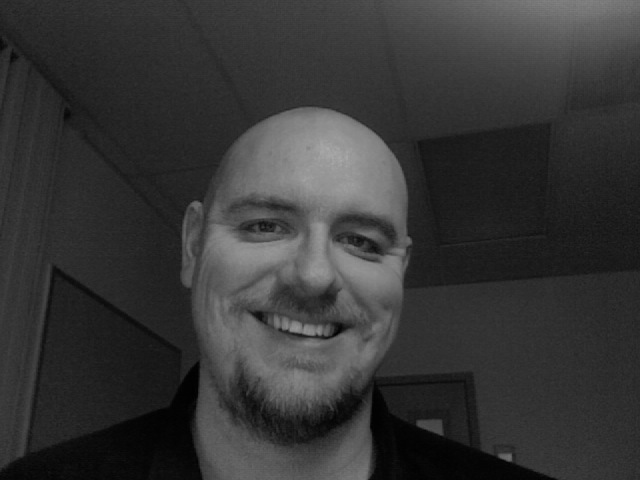## Hans Fugal: Optimizing the Constant-Q Transform in Octave

Abstract The constant-Q transform calculates the log-frequency spectrum and is therefore better suited to many computer music tasks than the DFT, which computes the linear-frequency spectrum. The direct calculation of the constant-Q transform is computationally inefficient, and so the efficient constant-Q transform algorithm is often used. The constant-Q transform and the efficient constant-Q transform algorithm are implemented and benchmarked in Octave. The efficient algorithm is not faster than the direct algorithm for a low minimum frequency. Additional vectorization of the algorithm is slower under most circumstances, and using sparse matrices gives marginal speedup.Paper: PDF
Slides: HansFugal_OptimizingConstantQ.pdf
Video Recording: Not available since presentation was held remotely from New Mexico
Homepage: http://hans.fugal.net/research/cq-octave